## research papersBIOLOGICALCRYSTALLOGRAPHY
ISSN: 1399-0047
Volume 57| Part 10| October 2001| Pages 1390-1396

## Patterson correlation methods: a review of molecular replacement with CNS

aLawrence Berkeley National Laboratory, One Cyclotron Road, Mail Stop 4-230, Berkeley, CA 94720, USA
*Correspondence e-mail: rwgrosse-kunstleve@lbl.gov

(Received 5 April 2001; accepted 12 June 2001)

This paper presents a review of the principles of molecular replacement with the audience of the CCP4 Study Weekend in mind. A complementary presentation with animated Patterson maps is available online (http://cci.lbl.gov/~rwgk/ccp4sw2001/ ). The implementation of molecular-replacement methods in the Crystallography and NMR System (CNS) is presented and discussed in some detail. The three principal components are the direct rotation function, Patterson correlation refinement and the fast translation function. CNS is available online and is free of charge for academic users.

### 1. Introduction

The method of molecular replacement was pioneered four decades ago by Hoppe (1957) and Rossmann & Blow (1962). The latter publication marks the beginning of practical application to the solution of macromolecular crystal structures. The term molecular replacement' is somewhat misleading because nothing is replaced' (but it is helpful for remembering the initials of one of the main champions of the method). The conventional understanding of what molecular replacement encompasses is the placement of one or more known molecular models in the unit cell of the crystal under study. The search models are often extracted from databases such as the Protein Data Bank (Berman et al., 2000) or different crystal forms that were solved previously.

In general, placing a molecular model in a unit cell is a six-dimensional search problem. The six degrees of freedom are most conveniently parameterized as three rotation angles and three translations along the basis vectors of the coordinate system. Conventionally, an asymmetric unit (a volume of the search space that is unique under symmetry) is sampled on a uniform grid. For a typical macromolecular unit cell, the product of angular and translational sampling points is usually too large to carry out an exhaustive six-dimensional search in a reasonable time with current computing resources. However, there have been cases where an exhaustive search has been carried out in spite of the computational cost (Sheriff et al., 1999).

Rossmann & Blow (1962) showed that it is possible to break up the six-dimensional search into two consecutive three-dimensional searches: a search for the angular orientation of the search molecule (rotation search) and a subsequent search for the translation (translation search). This greatly reduces the demand for computing resources. The total number of sampling points for the two three-dimensional searches is roughly proportional to the square root of the number of sampling points for the exhaustive six-dimensional search.

In the Crystallography and NMR System (CNS; Brunger et al., 1998), a third powerful procedure is usually inserted between the rotation search and the translation search: Patterson correlation refinement of the molecular orientation. We will discuss all three stages in the order in which they are typically used.

### 2. Rotation search

CNS implements two types of rotation search. We will refer to the first type as the traditional rotation search'. The second type is commonly referred to as the direct rotation search'. There is a conceptual distinction between these two types of rotation searches. In the traditional rotation search, two Patterson maps are rotated with respect to each other and then superimposed. This can be performed either in direct or in reciprocal space (Crowther, 1972; Navaza, 1987). In contrast, in a direct rotation search the molecular model is rotated directly. The term directly' is used because it is the fundamental concept of the rotation search to rotate the model. The following sections explain that rotating maps instead is actually a non-trivial optimization, devised to reduce the computer time.

#### 2.1. Principles of the rotation search

An animation that illustrates the general ideas behind the traditional and the direct rotation searches is available at http://cci.lbl.gov/~rwgk/ccp4sw2001/ . The fundamental prerequisites for the understanding of the methods are as follows.

 (i) An observed Patterson map can be directly computed from the experimental diffraction intensities by Fourier transformation. (ii) A model Patterson map can be directly computed from the oriented and translated search model and compared with the observed Patterson map by superposition. (iii) The peaks in a Patterson map correspond to the interatomic vectors of the crystal structure (Buerger, 1959).
To aid the interpretation of a Patterson map, the interatomic vectors can be classified as intramolecular (within a molecule) and intermolecular (between molecules). The intermolecular vectors for a given molecule can in turn be classified as vectors between the following.
 (i) Copies of the molecule arising from lattice translations. (ii) Copies of the molecule arising from rotational symmetry operations (note that mirror planes are improper' rotations and are included). (iii) Copies of the molecule arising from non-crystallographic symmetry (i.e. between molecules of the same kind). (iv) Other molecules of a different kind.
In the observed Patterson map, peaks arising from all of these different types of interatomic vectors are present. However, at the stage of the rotation search this is not true for the model Patterson map. Typically, only one search molecule is used at a time [see Tong & Rossmann (1990) for an alternative procedure that makes use of non-crystallographic symmetry at this stage], which eliminates any interatomic vectors arising from non-crystallographic symmetry or from other molecules of a different type (types iii and iv in the list above). Furthermore, the three translations that shift the search molecule to the correct location with respect to the rotational symmetry operations are unknown and the interatomic vectors arising from this symmetry (type ii in the list above) are best ignored. This is achieved by placing the search molecule in a P1 unit cell; in other words, by ignoring the rotational symmetry. Typically, it is computationally most efficient to place the search molecule with its center of gravity at the origin of the unit cell.

In summary, the model Patterson map has only peaks arsing from intramolecular vectors and intermolecular vectors between copies arising from lattice translations (type i in the list above). Conceptually, both the traditional and the direct rotation search superimpose this partial' Patterson map with the observed Patterson map. This can be viewed as a pattern-matching procedure. The model Patterson map is the search pattern. The observed Patterson map contains the search pattern in an unknown angular orientation. In the observed Patterson map, the search pattern is obscured by other patterns (other types of interatomic vectors) that are not considered in the model Patterson, and noise.

The traditional and the direct rotation search are two implementations of this pattern-matching concept. Both have their advantages and disadvantages.

In the direct rotation search, the model is rotated directly and a structure-factor calculation is carried out for each sampled angular orientation. This has the advantage of avoiding approximations such as interpolations, but the disadvantage of being computationally expensive.

In the traditional rotation search, the computationally expensive structure-factor calculation is carried out only once to obtain a model Patterson map (the next paragraph explains this in detail) which is then rotated and superimposed with the observed Patterson map. This has the advantage of being relatively fast, but the disadvantage of involving approximations.

Rotating Patterson maps with respect to each other is not as straightforward as it might seem at first sight. The problem arises from the fact that the two types of interatomic vectors present in the model Patterson map, the intramolecular vectors and the intermolecular vectors arising from lattice translations, are, in general,spatially intermixed. As the search model is rotated, vectors in the map that are close to each other rotate around different origins. In order to be able to consistently rotate the search pattern present in the map, the two types of vectors need to be spatially separated. Fig. 1explains how this can be achieved by placing the search model in an artificially enlarged unit cell. The intramolecular vectors in the large unit cell are then concentrated around the origin of the Patterson map and the intermolecular vectors are concentrated around the other lattice points. It is now possible to cut out the spherical region around the origin that contains the isolated intramolecular vectors, rotate it and superimpose the observed Patterson map in order to find the angular orientation with the best match. For reasons that will become apparent in the next section (equation 1), the spherical region is commonly referred to as the integration sphere. Obviously, the radius of the integration sphere is chosen to be similar to the largest intermolecular vector. In several implementations of the traditional rotation function, including CNS, a region around the large Patterson origin peak is normally omitted to improve the signal-to-noise ratio. The resulting actively used region of the model Patterson map is then called the integration shell (represented as a yellow region in Fig. 1d).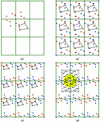Figure 1 This figure illustrates some of the difficulties involved in rotating the Patterson map calculated from a search molecule instead of rotating the search molecule directly. Each of the four figures shows nine (3 × 3) unit cells. Since the unit-cell parameters of crystal space and Patterson space are identical, they are superimposed in (a), (b) and (c) for convenience. (a) shows a simple molecule with four atoms (black) and the corresponding Patterson peaks (red) associated with one lattice point. (b) shows the molecule and the corresponding configuration of Patterson peaks translated to each lattice point. An alternating red–blue coloring scheme is used to distinguish the groups of Patterson peaks associated with each lattice translation. It can be seen that the groups are not spatially separated. Therefore, as the search model is rotated, vectors in the map that are close to each other rotate around different origins. In order to be able to consistently rotate the search pattern present in the map, the two types of vectors must be spatially separated. (c) shows the molecule and the corresponding Patterson peaks in an artificially enlarged unit cell. (For the purpose of this illustration, the molecule is scaled down instead of enlarging the entire drawing.) By making the unit cell sufficiently large, the groups of Patterson peaks associated with each lattice point are spatially separated. In (d) the molecule is omitted and the observed Patterson map superimposed. (Note that the observed Patterson map has the true unit-cell parameters of the crystal, while the model Patterson map is artificially enlarged.) The rotation function is computed by rotating the Patterson maps with respect to each other. For each sampling point in angular space, the correlation integral (1) is computed in the yellow integration shell.

#### 2.2. Rotation search target functions

In CNS, the traditional rotation function is evaluated in real space (Brünger, 1990). We will therefore use this term from now on. For the real-space rotation search, the Patterson correlation Rot(Ω) for a given angular orientation Ω is evaluated as the correlation integral,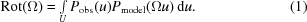Pobs and Pmodel are the observed and model Patterson functions, respectively, and u is a location vector in Patterson space U.

The direct rotation function CC(Ω) for a given angular orientation Ω of the search model is typically evaluated as the standard linear correlation coefficient of the observed and calculated normalized structure-factor amplitudes |E|2. The standard linear correlation coefficient is well known and frequently used in statistics to measure the strength of a linear relation of two variables. The formula for the evaluation of the correlation coefficient is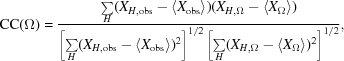where X = |E|2. The summations are computed for all Miller indices H. 〈X〉 denotes the mean of the XH.

At first sight, (1)and (2)look very different. However, in practice (1)is evaluated as the sum of products. (2)is again a sum of products. The difference is just that in (2)each variable is centered around its mean (this is achieved by the sub-expressions of the type X − 〈X〉) and the sums in the denominator normalize the coefficient such that it has values in the range from −1 to 1. In the absence of approximations, the two ways of evaluating the Patterson correlation should give essentially identical results, even though one is evaluated in real space and the other in reciprocal space. The absolute values will be different because the first expression is not normalized, but the rotation functions should be very similar except for a scaling factor.

#### 2.3. Comparison of rotation searches

The approximate relative CPU times for rotation searches with AMoRe (evaluated in reciprocal space; Navaza, 1987), the CNS real space and the CNS direct rotation function are shown in Table 1.

 Table 1Relative rotation-search CPU times (s)
 HyHEL-5 (26–10) Fab–digoxin complex (DeLano & Brünger, 1995).
 AMoRe 1 CNS real space 20 CNS direct 300

The direct rotation search is more than one order of magnitude slower than the real-space rotation search and AMoRe is yet another order of magnitude faster. What benefit can be expected from the direct rotation search in return for the large increase in computational expense?

In the previous section we stated that the two ways of evaluating the Patterson correlation should give similar results in the absence of approximations. However, in practice two significant approximations are made for the real-space rotation function. When the rotated Patterson map is superimposed with the observed Patterson map, grid points do not in general superimpose directly and interpolation has to be used. The other significant approximation is that the correlation integral (1) is only evaluated for a selected set of Patterson function peaks. Typically, only the highest 3000 peaks in the observed Patterson map are considered in the calculation. In contrast, the direct rotation function is evaluated uniformly for the entire unit cell and does not involve interpolations.

DeLano & Brünger (1995) systematically compared the signal-to-noise ratio for a number of test cases. They define the signal-to-noise ratio of rotation functions as the ratio of the value of the highest signal point to that of the highest noise point, measured in standard deviations above the mean. Points' of the rotation function are defined as peaks that are left after reduction by spatial cluster analysis (DeLano & Brünger, 1995). A `signal' is defined by the radius of convergence of Patterson correlation refinement (see §3). Empirical observation led DeLano & Brünger (1995) to the conclusion that a rotation-function peak that is within about 15° of one of the correct orientations will, in general, converge to it by Patterson correlation refinement. Rotation-function peaks that are within the 15° range were thus considered to be a signal. Points outside this range were considered to be noise.

A typical result of DeLano and Brünger's systematic comparisons is shown in Fig. 2for search models with all atoms, a polyalanine chain and just the Cα atoms. The direct rotation function consistently has a much better signal-to-noise ratio. Similarly, in Fig. 3the high-resolution limit is varied. Again, the direct rotation function consistently has a significantly better signal-to-noise ratio compared with the real-space rotation functions, both with and without removal of the Patterson origin peak.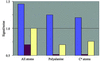Figure 2 A comparison of rotation functions for HyHEL-5 (26–10) Fab–digoxin complex at 15–4 Å resolution (DeLano & Brünger, 1995). Lilac, direct; burgundy, real space; yellow, real space, origin-subtracted.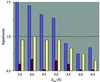Figure 3 A comparison of rotation functions for HyHEL-5 (26–10) Fab–digoxin complex (DeLano & Brünger, 1995). Lilac, direct; burgundy, real space; yellow, real space, origin-subtracted.

### 3. Patterson correlation refinement

The second stage of the CNS molecular-replacement procedure is Patterson correlation (PC) refinement, which is the intervening step between the rotation search and the translation search (Brünger, 1990). The goal of PC refinement is to improve the overall orientation of the search model. Typically, the refinement is carried out for rigid bodies such as domains, subdomains or secondary-structure elements. The major difference from normal crystallographic rigid-body refinement is that PC refinement is conducted without using crystallographic symmetry. The rationale for this is similar to that for not using the symmetry in the rotation search (see §2.1). The target function of PC refinement is typically defined as the standard linear correlation between observed and calculated squared normalized structure-factor amplitudes (|E2|).

By improving the accuracy of the search model for the correct angular orientation, PC refinement improves the discrimination between correct and incorrect orientations and therefore enables the location of the correct peak in a noisy rotation function. In general, PC refinement makes the combination of a three-dimensional rotation search with a subsequent three-dimensional translation search much more robust, so that one does not have to resort to exhaustive six-dimensional searches.

Brünger (1997) systematically studied the radius of convergence of rigid-body PC refinement under various conditions. One of the examples is a structure with two domains that are connected by a linker region. One domain was kept stationary and the other was systematically misaligned. Fig. 4shows the value of the Patterson correlation coefficient after PC refinement as a function of the initial misaligned interdomain angle. In this particular case, it is found that the PC refinement converges back to the correct angle if the second domain is misaligned by up to approximately 13°.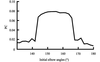Figure 4 The radius of convergence of PC refinement of AN02 at 15–4 Å resolution (Brünger, 1997). The horizontal axis is centered at the correct elbow angle (155°). The vertical axis shows the value of PC after PC refinement.

Another way to assess the power of PC refinement is shown in Fig. 5. This figure shows that pre-translation PC refinement has the potential to drastically reduce the number of noise peaks in the translation function. Owing to this noise reduction it can often become immediately obvious what the correct position of the search molecule is.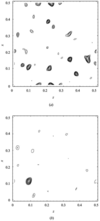Figure 5 A comparison of translation searches (a) without and (b) with prior PC refinement of the interdomain angles and positions of the HyHEL5 Fab search model using data between 15 and 4 Å resolution (Brünger, 1997). (a) Without PC refinement there are many additional noise peaks which obscure the true translation solution. (b) With PC refinement the noise peaks are greatly diminished in size and the true translation solution is readily identified.

### 4. Translation search

At this stage, the angular orientation of the search molecule is assumed to be known. The remaining problem is to determine the location of this oriented search molecule with respect to the symmetry elements. The fundamental concept for solving this problem is straightforward: the unit cell is subdivided into a regular grid and the search molecule is moved to each grid point in turn. At each location, a structure-factor calculation is performed. The agreement between these calculated and the observed structure factors is evaluated by some type of target function. Depending on the space-group symmetry, for macromolecules the result is a two- or three-dimensional translation function similar to the example shown in Fig. 5.

The translation-search target functions available in CNS include the standard linear correlation coefficient (equation 2modified for translation instead of angular orientation) of normalized or unnormalized structure-factor amplitudes, both squared and unsquared (|E|, |E|2, |F|, |F|2) and the crystallographic R factor. The use of the latter is complicated by the fact that a reasonably accurate estimate of the scale factor between observed and calculated structure factors is required. The literature contains no conclusive evidence that this is a significant disadvantage in practice. However, a correlation coefficient is the default choice in CNS.

Computing a translation function is relatively time-consuming and optimizations are essential. Fujinaga & Read (1987) introduced an efficient method for computing the structure factors for each sampling point. More recently, Navaza & Vernoslova (1995) introduced an ingenious fast Fourier transform based method for computing the final two- or three-dimensional translation function without explicitly computing the structure factors as intermediate results. The target function for this fast translation function is the correlation coefficient between squared structure-factor amplitudes (|F|2).

Both the more conventional Fujinaga & Read (1987) type translation function and the fast translation function are implemented in CNS. Table 2shows a comparison of the run times of the CNS conventional translation function (CTF) and the CNS fast translation function (FTF) for a variety of symmetries, unit-cell sizes and resolution ranges. The rightmost column of Table 2shows the factor by which the fast translation function is faster than the conventional one. Depending on the symmetry, unit-cell dimensions and resolution range, the fast translation function is 200 to almost 500 times faster than the conventional search.

 Table 2CPU times for a conventional translation function (CTF) and the fast translation function (FTF) for several test cases with different unit-cell sizes and symmetries (Grosse-Kunstleve & Brunger, 1999)
Space group Unit-cell parameters (Å) dmin (Å) Time CTF (s) Time FTF (s) Factor
P212121 a = 65.5, b = 72.2, c = 45.0 4 245 0.8 306
C2221 a = 42.1, b = 97.1, c = 91.9 3 1700 8 210
C2221 a = 64.1, b = 102.0, c = 187.0 4 3000 13 230
C222 a = 91.9, b = 168.0, c = 137.8 4.5 7850 17 460
P4332 a = 272.8 6 1129644 2400 470

Because of this enormous increase in speed, the fast translation function has also found a use in the automatic heavy-atom search procedure in CNS (Grosse-Kunstleve & Brunger, 1999). The search procedure consists primarily of alternating single-atom translation functions and PC refinements. This strategy is only practical if the fast translation function is used. CNS has been used by independent groups to automatically locate up to 40 heavy-atom sites in the asymmetric unit (Walsh et al., 2000).

### 5. Summary

The CNS procedures that are presented in the previous sections can be combined into a powerful general strategy for solving difficult molecular-replacement problems.

 (i) Direct rotation searches of model domains. The systematic investigation of DeLano & Brünger (1995) shows that the direct rotation search has a high chance of finding the correct solutions among the highest ranked points in the rotation function (after reduction by spatial cluster analysis) and has the ability to produce a recognizable signal even for relatively small subunits (Figs. 1and 2). (ii) PC refinement of the overall orientation and the interdomain angles. PC refinement enhances the discrimination between correct and incorrect rotation-function points by improving the search models that are within 10–15° of the correct angular orientation (Fig. 4). Another consequence of the improved model quality is that the signal-to-noise ratio in the subsequent translation function is enhanced (Fig. 5). (iii) Fast translation function. The implementation of the translation function of Navaza & Vernoslova (1995) in CNS is fast enough to be applied to a large number of putative rotation-function solutions (e.g. testing 100 solutions is entirely feasible for typical macromolecular structures).
For routine molecular-replacement structure solutions, a highly optimized traditional rotation search as is implemented in the AMoRe program (Navaza, 1994) will give the correct answer much faster than the direct rotation search in CNS. However, for more difficult cases, the unique combination of enhanced signal-to-noise ratio, spatial cluster analysis of the rotation function peaks, PC refinement and the fast translation function is a very attractive and much faster alternative when compared with full six-dimensional searches.

The time needed for the computation of the direct rotation function could be substantially reduced by using a well known optimization employed by several other programs (Castellano et al., 1992; Kissinger et al., 1999; Glykos & Kokkinidis, 2000). In CNS, a full structure-factor calculation is carried out for each sampling point in the direct rotation search. Alternatively, a fine sampling of the molecular transform and interpolation in reciprocal space could be employed. We expect that the resulting fast direct rotation search will be at least an order of magnitude faster. Therefore, the general strategy outlined above will be even more practical.

### 6. Program availability

CNS is available online at http://cns.csb.yale.edu/ and is free of charge for academic users. The procedures that are discussed in this paper are implemented in the two standard input files cross_rotation.inp (real-space rotation search and direct rotation search) and translation.inp (PC refinement, conventional translation function and fast translation function).

### References

© International Union of Crystallography. Prior permission is not required to reproduce short quotations, tables and figures from this article, provided the original authors and source are cited. For more information, click here.BIOLOGICALCRYSTALLOGRAPHY
ISSN: 1399-0047
Volume 57| Part 10| October 2001| Pages 1390-1396
Follow Acta Cryst. D